# RD Sharma Solutions for Class 10 Maths Chapter 7 Statistics Exercise 7.3

This exercise exposes students to find the arithmetic mean of a continuous frequency distribution. The RD Sharma Solutions Class 10 provides a stepwise solution to problems so that students attain strong conceptual knowledge. All the solutions are on par with the latest CBSE pattern. Further, students can access the RD Sharma Solutions for Class 10 Maths Chapter 7 Statistics Exercise 7.3, PDF provided below.

## RD Sharma Solutions for Class 10 Maths Chapter 7 Statistics Exercise 7.3 Download PDF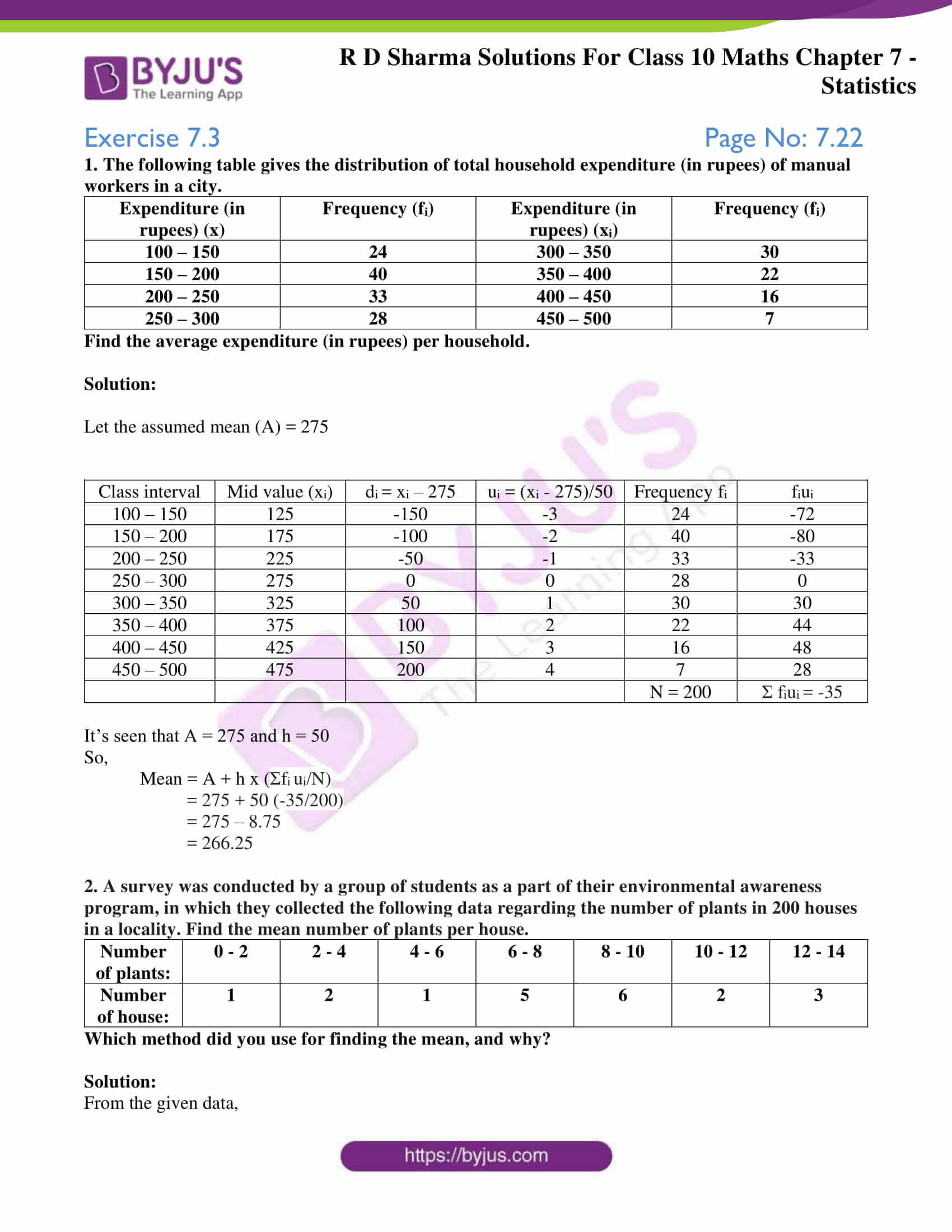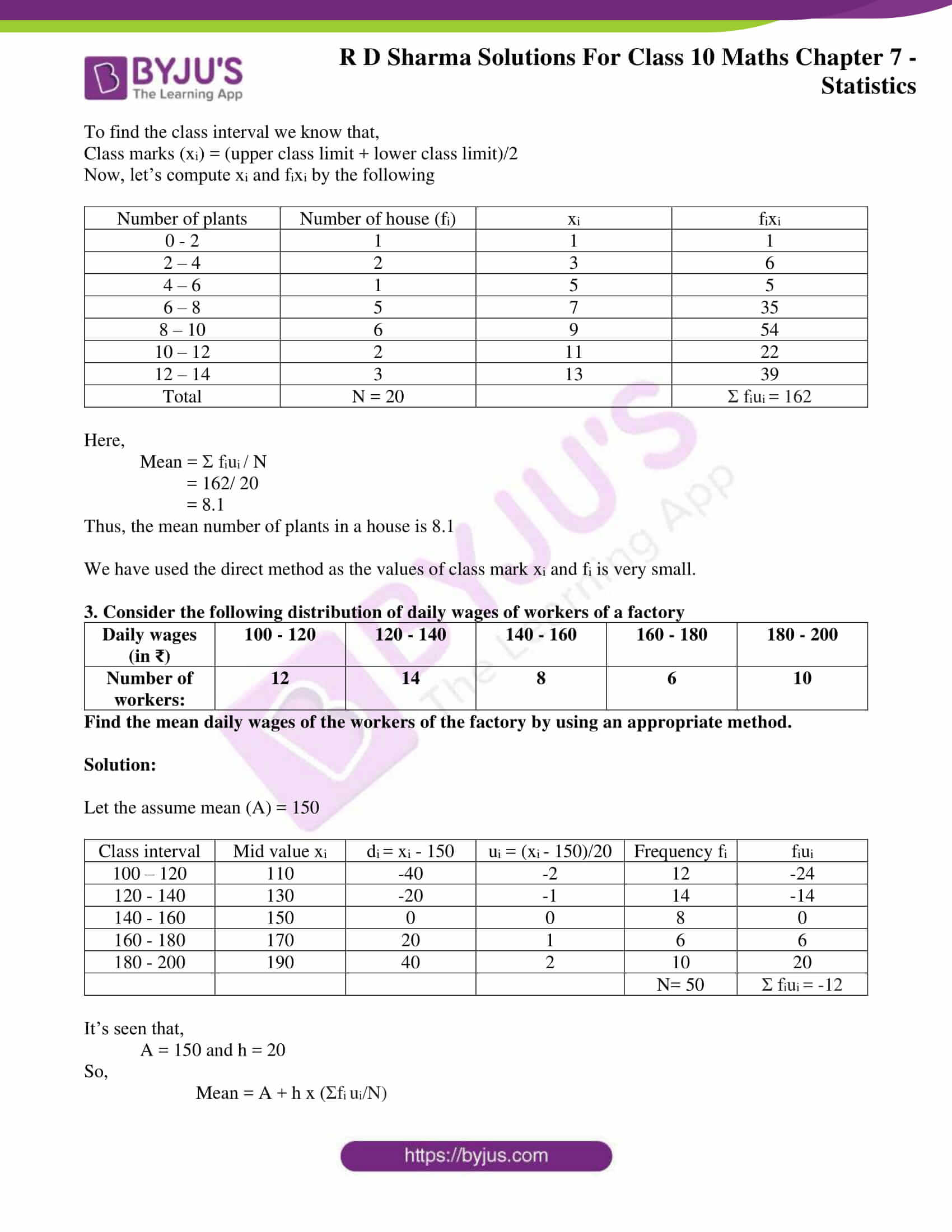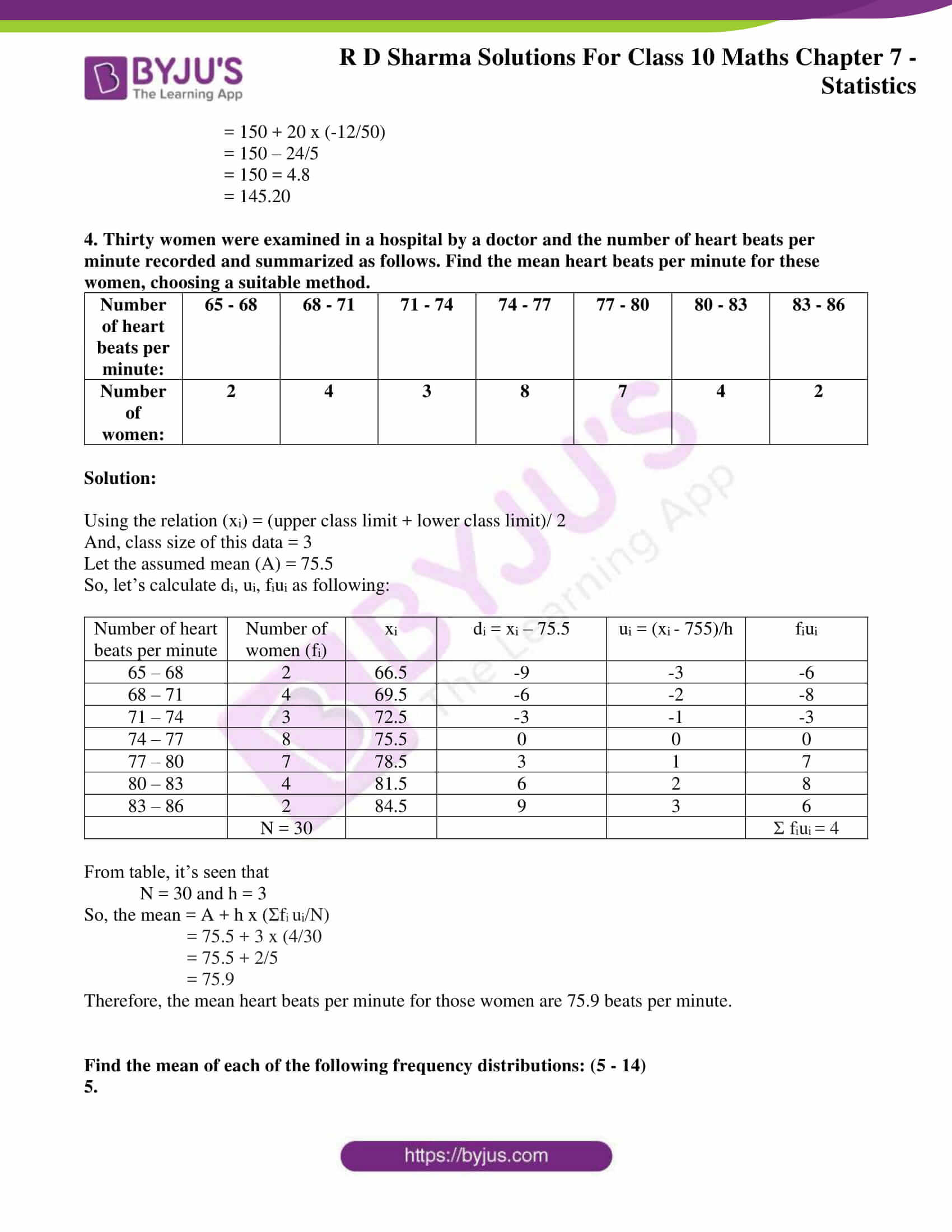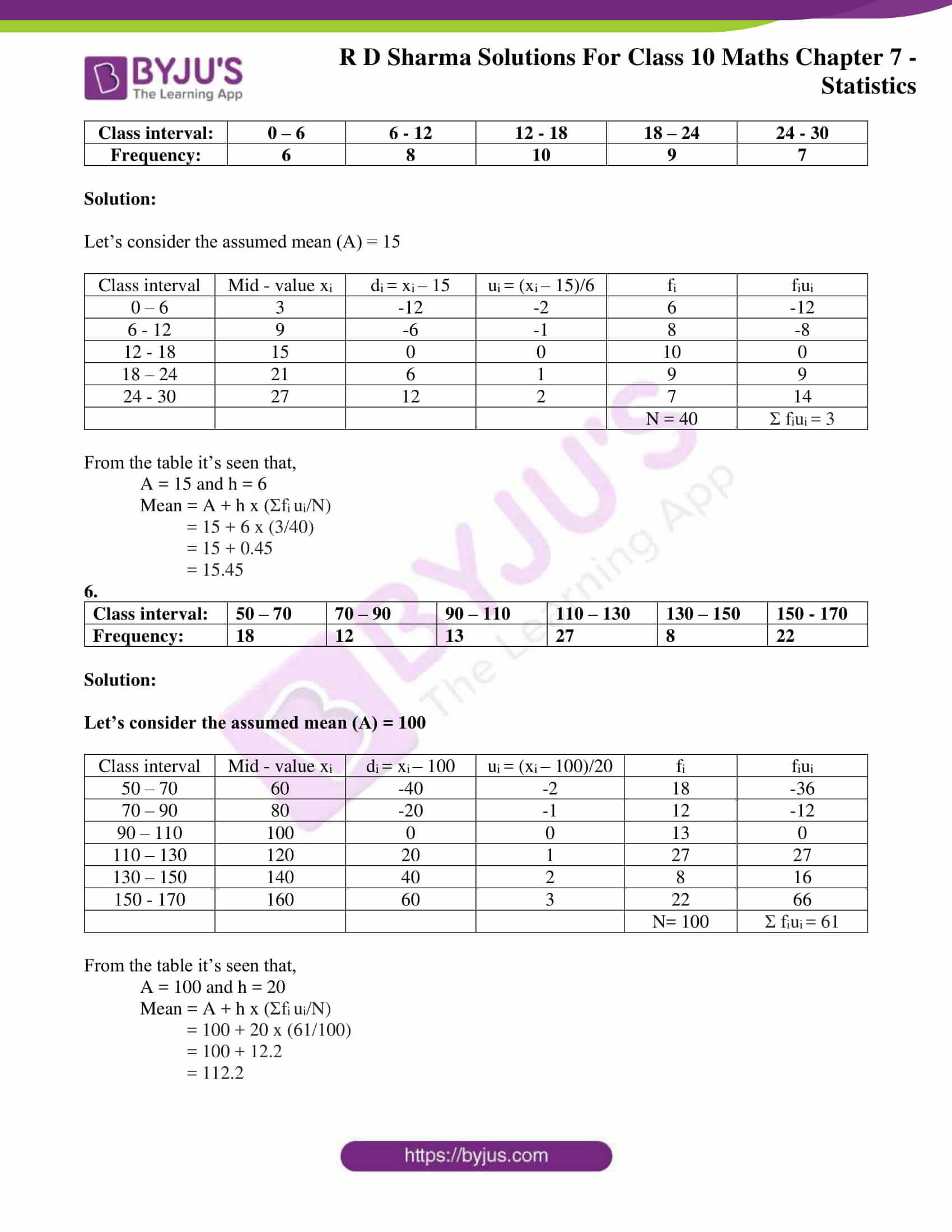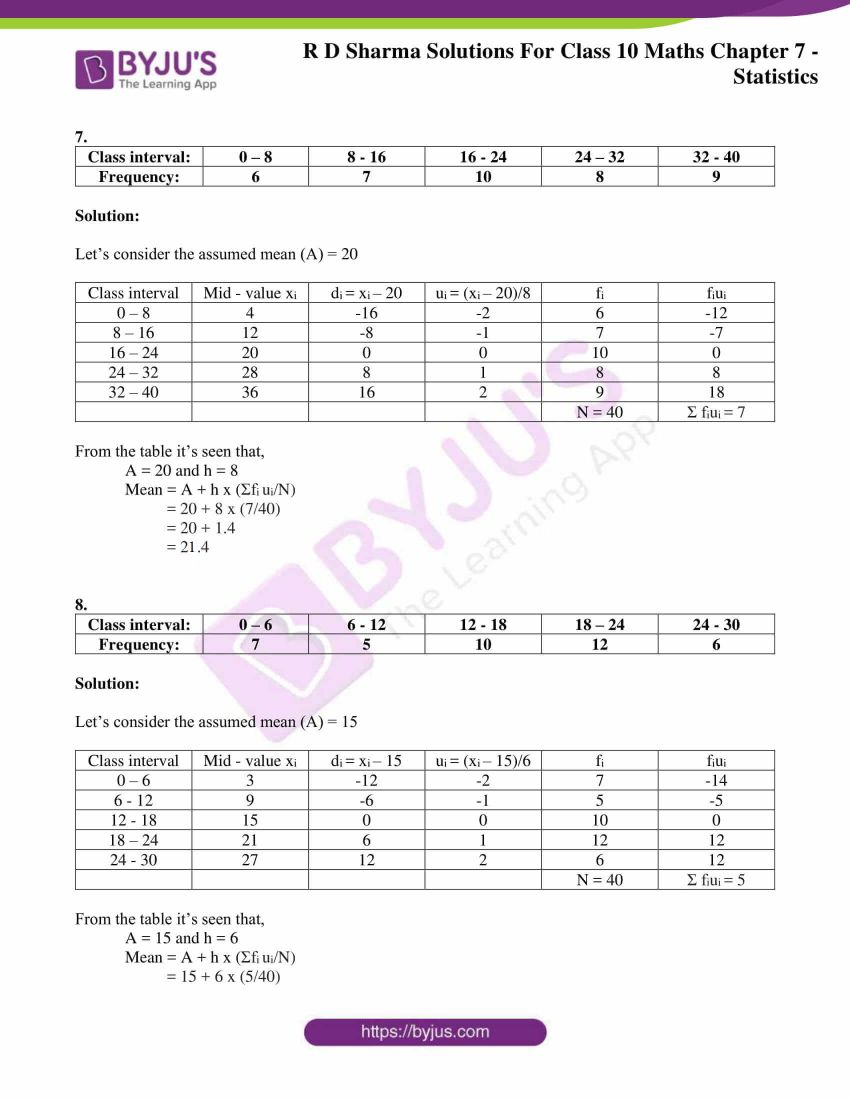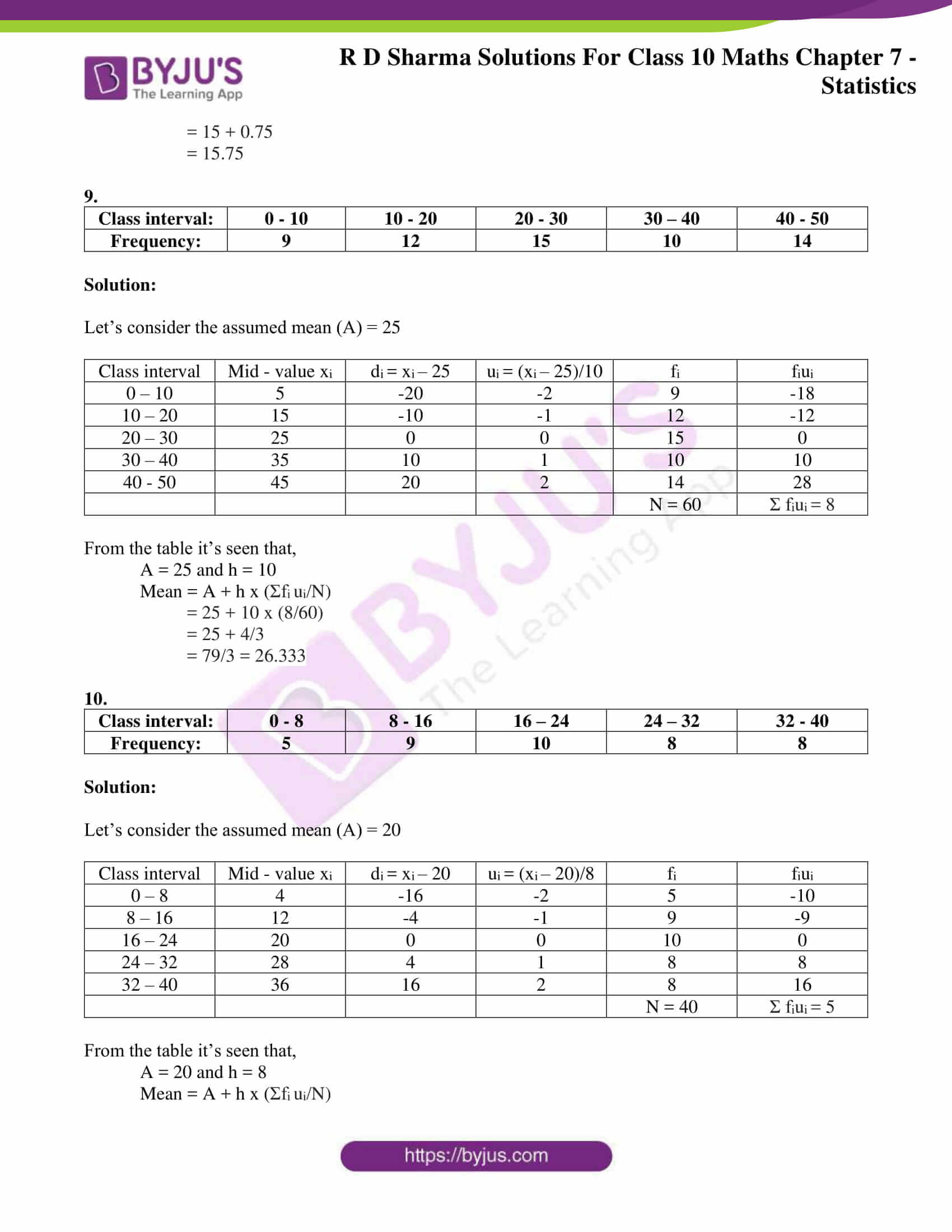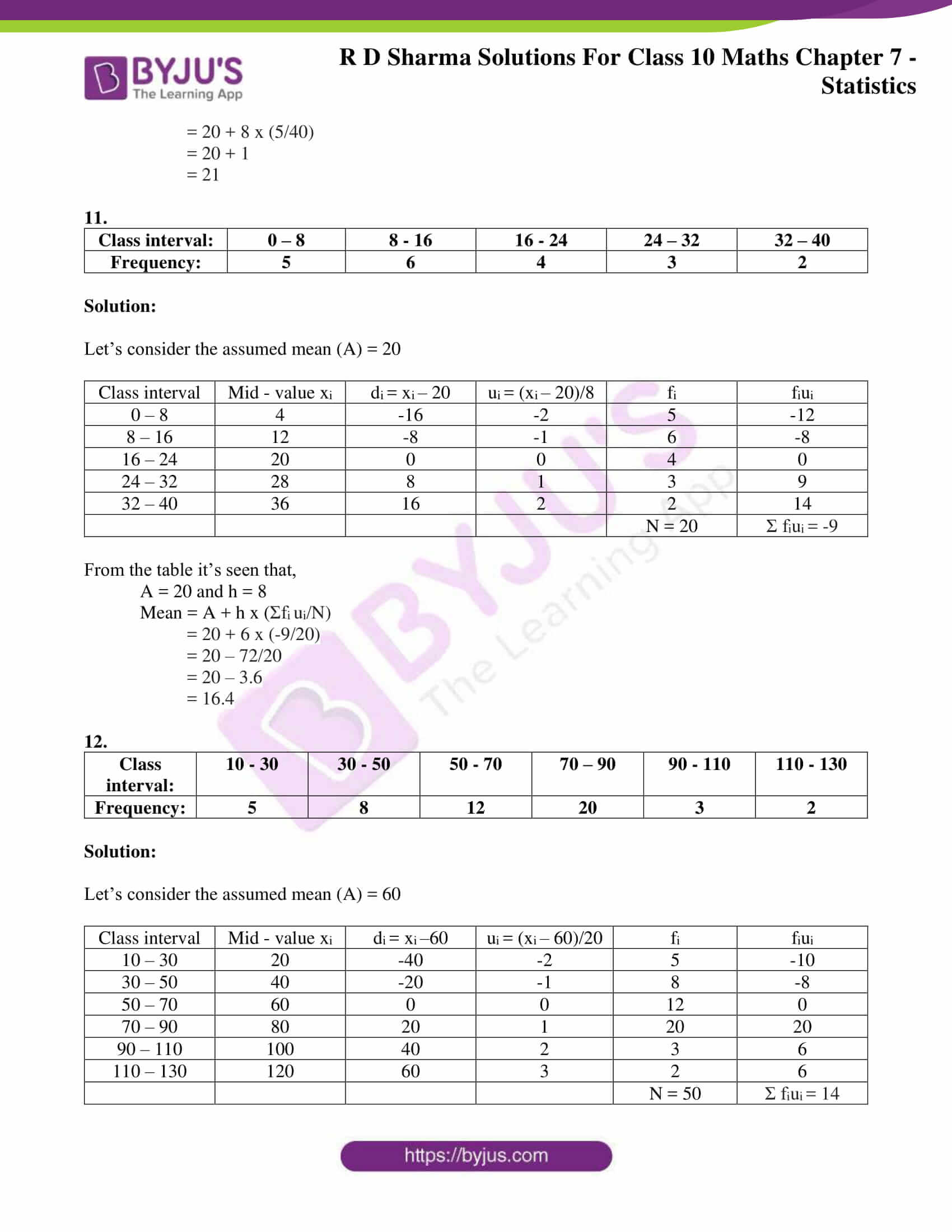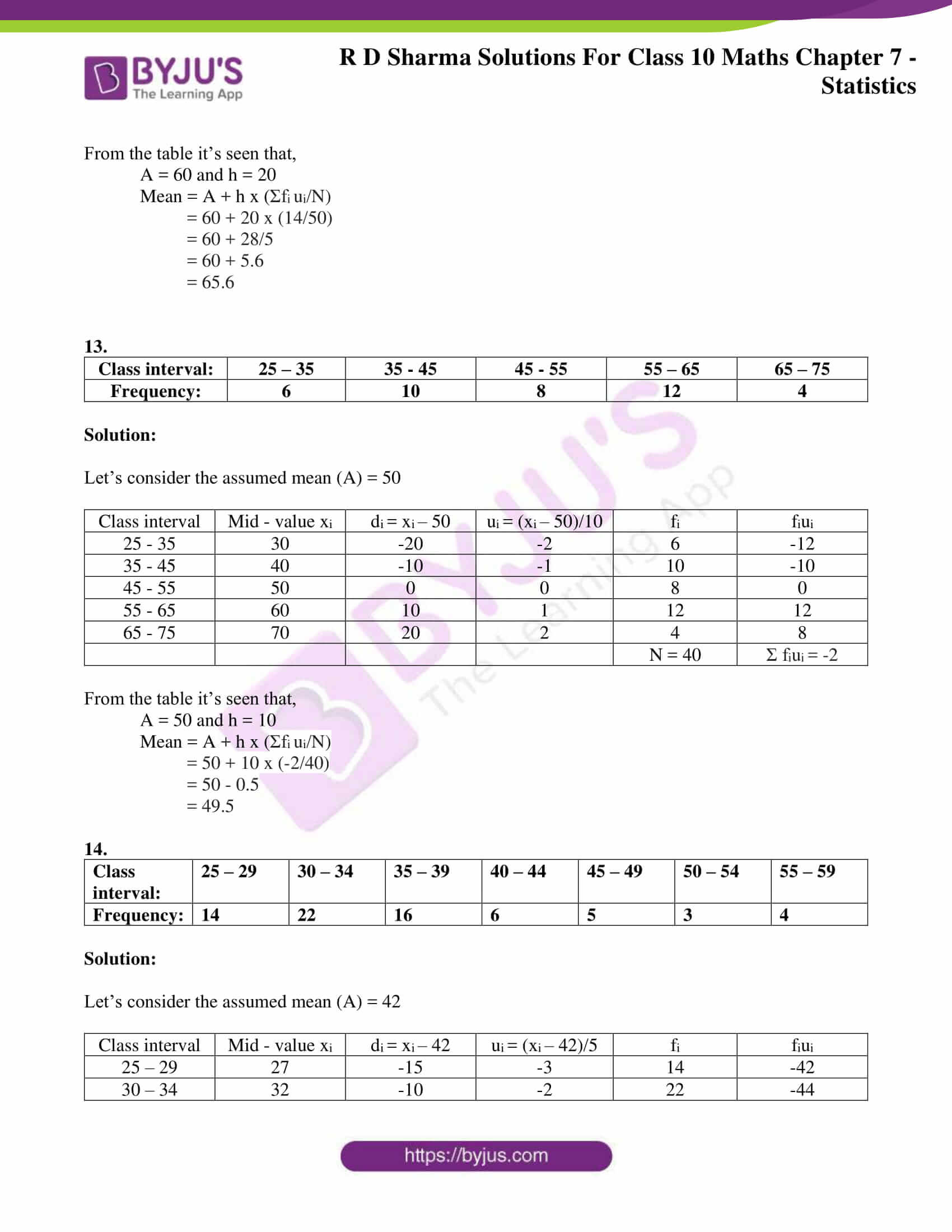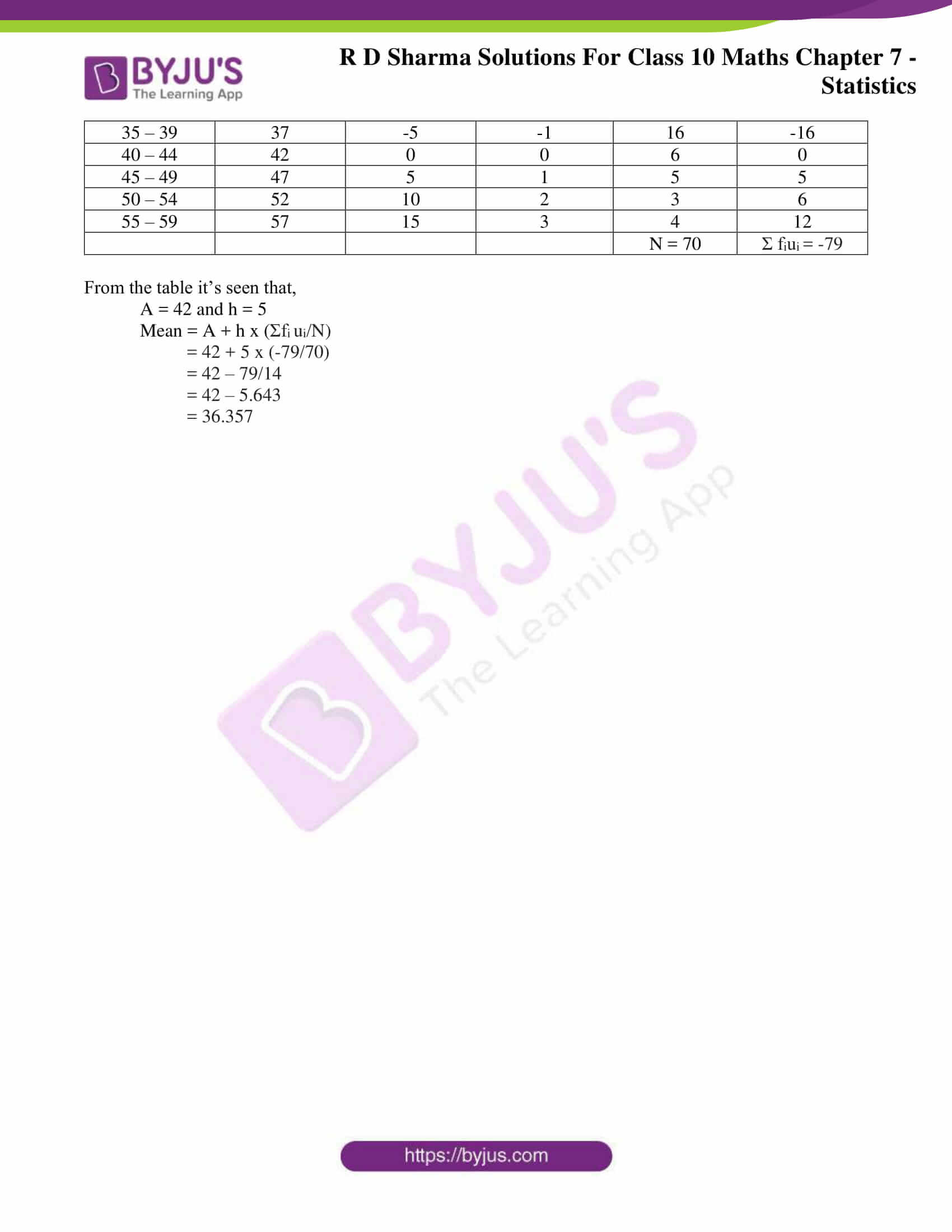### Access RD Sharma Solutions for Class 10 Maths Chapter 7 Statistics Exercise 7.3

1. The following table gives the distribution of total household expenditure (in rupees) of manual workers in a city.

 Expenditure (in rupees) (x) Frequency (fi) Expenditure (in rupees) (xi) Frequency (fi) 100 – 150 24 300 – 350 30 150 – 200 40 350 – 400 22 200 – 250 33 400 – 450 16 250 – 300 28 450 – 500 7

Find the average expenditure (in rupees) per household.

Solution:

Let the assumed mean (A) = 275

 Class interval Mid value (xi) di = xi – 275 ui = (xi – 275)/50 Frequency fi fiui 100 – 150 125 -150 -3 24 -72 150 – 200 175 -100 -2 40 -80 200 – 250 225 -50 -1 33 -33 250 – 300 275 0 0 28 0 300 – 350 325 50 1 30 30 350 – 400 375 100 2 22 44 400 – 450 425 150 3 16 48 450 – 500 475 200 4 7 28 N = 200 Σ fiui = -35

It’s seen that A = 275 and h = 50

So,

Mean = A + h x (Σfi ui/N)

= 275 + 50 (-35/200)

= 275 – 8.75

= 266.25

2. A survey was conducted by a group of students as a part of their environmental awareness program, in which they collected the following data regarding the number of plants in 200 houses in a locality. Find the mean number of plants per house.

 Number of plants: 0 – 2 2 – 4 4 – 6 6 – 8 8 – 10 10 – 12 12 – 14 Number of house: 1 2 1 5 6 2 3

Which method did you use for finding the mean, and why?

Solution:

From the given data,

To find the class interval we know that,

Class marks (xi) = (upper class limit + lower class limit)/2

Now, let’s compute xi and fixi by the following

 Number of plants Number of house (fi) xi fixi 0 – 2 1 1 1 2 – 4 2 3 6 4 – 6 1 5 5 6 – 8 5 7 35 8 – 10 6 9 54 10 – 12 2 11 22 12 – 14 3 13 39 Total N = 20 Σ fiui = 162

Here,

Mean = Σ fiui / N

= 162/ 20

= 8.1

Thus, the mean number of plants in a house is 8.1

We have used the direct method as the values of class mark xi and fi is very small.

3. Consider the following distribution of daily wages of workers of a factory

 Daily wages (in ₹) 100 – 120 120 – 140 140 – 160 160 – 180 180 – 200 Number of workers: 12 14 8 6 10

Find the mean daily wages of the workers of the factory by using an appropriate method.

Solution:

Let the assume mean (A) = 150

 Class interval Mid value xi di = xi – 150 ui = (xi – 150)/20 Frequency fi fiui 100 – 120 110 -40 -2 12 -24 120 – 140 130 -20 -1 14 -14 140 – 160 150 0 0 8 0 160 – 180 170 20 1 6 6 180 – 200 190 40 2 10 20 N= 50 Σ fiui = -12

It’s seen that,

A = 150 and h = 20

So,

Mean = A + h x (Σfi ui/N)

= 150 + 20 x (-12/50)

= 150 – 24/5

= 150 = 4.8

= 145.20

4. Thirty women were examined in a hospital by a doctor and the number of heart beats per minute recorded and summarized as follows. Find the mean heart beats per minute for these women, choosing a suitable method.

 Number of heart beats per minute: 65 – 68 68 – 71 71 – 74 74 – 77 77 – 80 80 – 83 83 – 86 Number of women: 2 4 3 8 7 4 2

Solution:

Using the relation (xi) = (upper class limit + lower class limit)/ 2

And, class size of this data = 3

Let the assumed mean (A) = 75.5

So, let’s calculate di, ui, fiui as following:

 Number of heart beats per minute Number of women (fi) xi di = xi – 75.5 ui = (xi – 755)/h fiui 65 – 68 2 66.5 -9 -3 -6 68 – 71 4 69.5 -6 -2 -8 71 – 74 3 72.5 -3 -1 -3 74 – 77 8 75.5 0 0 0 77 – 80 7 78.5 3 1 7 80 – 83 4 81.5 6 2 8 83 – 86 2 84.5 9 3 6 N = 30 Σ fiui = 4

From table, it’s seen that

N = 30 and h = 3

So, the mean = A + h x (Σfi ui/N)

= 75.5 + 3 x (4/30

= 75.5 + 2/5

= 75.9

Therefore, the mean heart beats per minute for those women are 75.9 beats per minute.

Find the mean of each of the following frequency distributions: (5 – 14)

5.

 Class interval: 0 – 6 6 – 12 12 – 18 18 – 24 24 – 30 Frequency: 6 8 10 9 7

Solution:

Let’s consider the assumed mean (A) = 15

 Class interval Mid – value xi di = xi – 15 ui = (xi – 15)/6 fi fiui 0 – 6 3 -12 -2 6 -12 6 – 12 9 -6 -1 8 -8 12 – 18 15 0 0 10 0 18 – 24 21 6 1 9 9 24 – 30 27 12 2 7 14 N = 40 Σ fiui = 3

From the table it’s seen that,

A = 15 and h = 6

Mean = A + h x (Σfi ui/N)

= 15 + 6 x (3/40)

= 15 + 0.45

= 15.45

6.

 Class interval: 50 – 70 70 – 90 90 – 110 110 – 130 130 – 150 150 – 170 Frequency: 18 12 13 27 8 22

Solution:

Let’s consider the assumed mean (A) = 100

 Class interval Mid – value xi di = xi – 100 ui = (xi – 100)/20 fi fiui 50 – 70 60 -40 -2 18 -36 70 – 90 80 -20 -1 12 -12 90 – 110 100 0 0 13 0 110 – 130 120 20 1 27 27 130 – 150 140 40 2 8 16 150 – 170 160 60 3 22 66 N= 100 Σ fiui = 61

From the table it’s seen that,

A = 100 and h = 20

Mean = A + h x (Σfi ui/N)

= 100 + 20 x (61/100)

= 100 + 12.2

= 112.2

7.

 Class interval: 0 – 8 8 – 16 16 – 24 24 – 32 32 – 40 Frequency: 6 7 10 8 9

Solution:

Let’s consider the assumed mean (A) = 20

 Class interval Mid – value xi di = xi – 20 ui = (xi – 20)/8 fi fiui 0 – 8 4 -16 -2 6 -12 8 – 16 12 -8 -1 7 -7 16 – 24 20 0 0 10 0 24 – 32 28 8 1 8 8 32 – 40 36 16 2 9 18 N = 40 Σ fiui = 7

From the table it’s seen that,

A = 20 and h = 8

Mean = A + h x (Σfi ui/N)

= 20 + 8 x (7/40)

= 20 + 1.4

= 21.4

8.

 Class interval: 0 – 6 6 – 12 12 – 18 18 – 24 24 – 30 Frequency: 7 5 10 12 6

Solution:

Let’s consider the assumed mean (A) = 15

 Class interval Mid – value xi di = xi – 15 ui = (xi – 15)/6 fi fiui 0 – 6 3 -12 -2 7 -14 6 – 12 9 -6 -1 5 -5 12 – 18 15 0 0 10 0 18 – 24 21 6 1 12 12 24 – 30 27 12 2 6 12 N = 40 Σ fiui = 5

From the table it’s seen that,

A = 15 and h = 6

Mean = A + h x (Σfi ui/N)

= 15 + 6 x (5/40)

= 15 + 0.75

= 15.75

9.

 Class interval: 0 – 10 10 – 20 20 – 30 30 – 40 40 – 50 Frequency: 9 12 15 10 14

Solution:

Let’s consider the assumed mean (A) = 25

 Class interval Mid – value xi di = xi – 25 ui = (xi – 25)/10 fi fiui 0 – 10 5 -20 -2 9 -18 10 – 20 15 -10 -1 12 -12 20 – 30 25 0 0 15 0 30 – 40 35 10 1 10 10 40 – 50 45 20 2 14 28 N = 60 Σ fiui = 8

From the table it’s seen that,

A = 25 and h = 10

Mean = A + h x (Σfi ui/N)

= 25 + 10 x (8/60)

= 25 + 4/3

= 79/3 = 26.333

10.

 Class interval: 0 – 8 8 – 16 16 – 24 24 – 32 32 – 40 Frequency: 5 9 10 8 8

Solution:

Let’s consider the assumed mean (A) = 20

 Class interval Mid – value xi di = xi – 20 ui = (xi – 20)/8 fi fiui 0 – 8 4 -16 -2 5 -10 8 – 16 12 -4 -1 9 -9 16 – 24 20 0 0 10 0 24 – 32 28 4 1 8 8 32 – 40 36 16 2 8 16 N = 40 Σ fiui = 5

From the table it’s seen that,

A = 20 and h = 8

Mean = A + h x (Σfi ui/N)

= 20 + 8 x (5/40)

= 20 + 1

= 21

11.

 Class interval: 0 – 8 8 – 16 16 – 24 24 – 32 32 – 40 Frequency: 5 6 4 3 2

Solution:

Let’s consider the assumed mean (A) = 20

 Class interval Mid – value xi di = xi – 20 ui = (xi – 20)/8 fi fiui 0 – 8 4 -16 -2 5 -12 8 – 16 12 -8 -1 6 -8 16 – 24 20 0 0 4 0 24 – 32 28 8 1 3 9 32 – 40 36 16 2 2 14 N = 20 Σ fiui = -9

From the table it’s seen that,

A = 20 and h = 8

Mean = A + h x (Σfi ui/N)

= 20 + 6 x (-9/20)

= 20 – 72/20

= 20 – 3.6

= 16.4

12.

 Class interval: 10 – 30 30 – 50 50 – 70 70 – 90 90 – 110 110 – 130 Frequency: 5 8 12 20 3 2

Solution:

Let’s consider the assumed mean (A) = 60

 Class interval Mid – value xi di = xi –60 ui = (xi – 60)/20 fi fiui 10 – 30 20 -40 -2 5 -10 30 – 50 40 -20 -1 8 -8 50 – 70 60 0 0 12 0 70 – 90 80 20 1 20 20 90 – 110 100 40 2 3 6 110 – 130 120 60 3 2 6 N = 50 Σ fiui = 14

From the table it’s seen that,

A = 60 and h = 20

Mean = A + h x (Σfi ui/N)

= 60 + 20 x (14/50)

= 60 + 28/5

= 60 + 5.6

= 65.6

13.

 Class interval: 25 – 35 35 – 45 45 – 55 55 – 65 65 – 75 Frequency: 6 10 8 12 4

Solution:

Let’s consider the assumed mean (A) = 50

 Class interval Mid – value xi di = xi – 50 ui = (xi – 50)/10 fi fiui 25 – 35 30 -20 -2 6 -12 35 – 45 40 -10 -1 10 -10 45 – 55 50 0 0 8 0 55 – 65 60 10 1 12 12 65 – 75 70 20 2 4 8 N = 40 Σ fiui = -2

From the table it’s seen that,

A = 50 and h = 10

Mean = A + h x (Σfi ui/N)

= 50 + 10 x (-2/40)

= 50 – 0.5

= 49.5

14.

 Class interval: 25 – 29 30 – 34 35 – 39 40 – 44 45 – 49 50 – 54 55 – 59 Frequency: 14 22 16 6 5 3 4

Solution:

Let’s consider the assumed mean (A) = 42

 Class interval Mid – value xi di = xi – 42 ui = (xi – 42)/5 fi fiui 25 – 29 27 -15 -3 14 -42 30 – 34 32 -10 -2 22 -44 35 – 39 37 -5 -1 16 -16 40 – 44 42 0 0 6 0 45 – 49 47 5 1 5 5 50 – 54 52 10 2 3 6 55 – 59 57 15 3 4 12 N = 70 Σ fiui = -79

From the table it’s seen that,

A = 42 and h = 5

Mean = A + h x (Σfi ui/N)

= 42 + 5 x (-79/70)

= 42 – 79/14

= 42 – 5.643

= 36.357